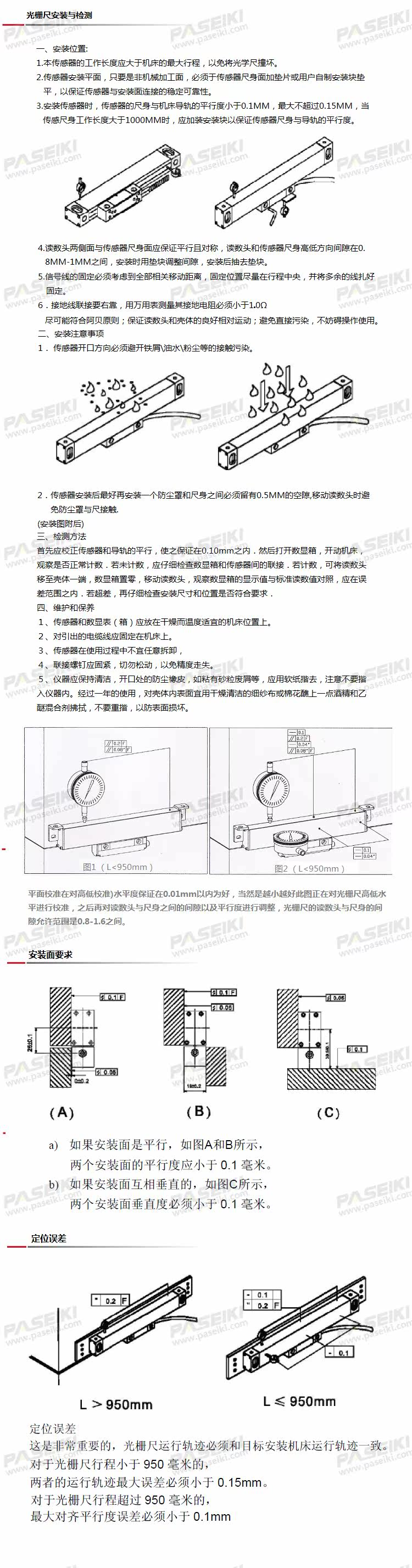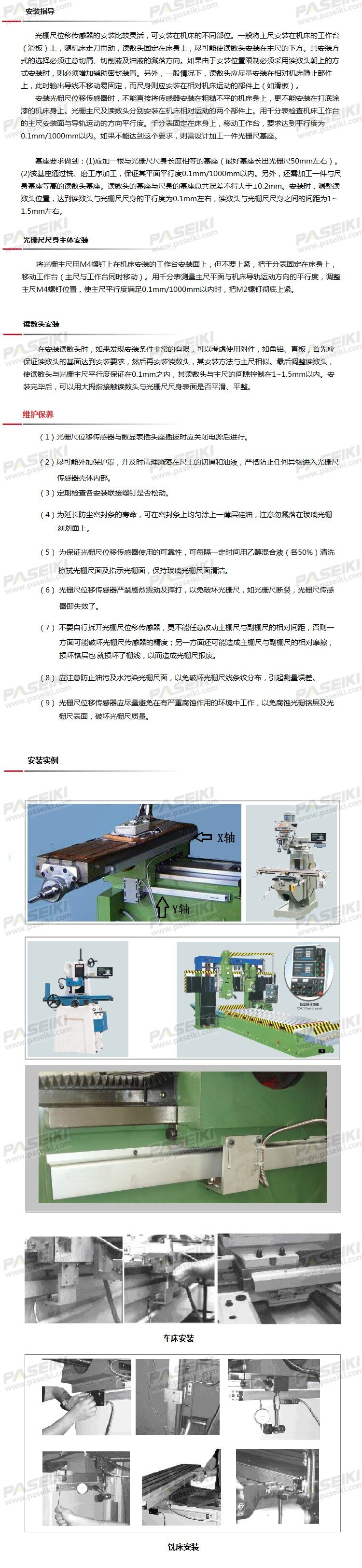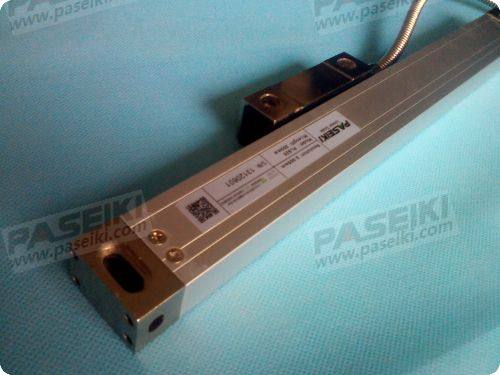### 高精度光栅尺

• 品牌   PASEIKI
• 型号   PLS25/21
• 描述

...

PLSEIKI光栅尺按外观大小分为：PLS2-H高精度系列光栅尺

>> PLS系列光栅尺输出信号及接线图【双层防护胶封设计】

【每支尺均通过激光校验】

>> 订购指南

 类别及量程 尺身型号 分辨率 零位 电源电压 输出方式 数据线长度 接头方式 PLS 2 05 C 5 L MST DB9 1=小型尺身 0.1=1μm N=无零位 5 = 5V L=线性输出 M01N=1m SC=无接头 2=通用尺身 0.5=5μm C=中间零位 24 = 24V P=PNP输出 M02/N=2m DB9=DB9 3=加固尺身 L=左零位 0528 =5~28V N=NPN输出 M10/N=10m 2P=PLC尺身 R=右零位 MST=标准线长